# RD Sharma Solutions for Class 8 Maths Chapter 21 Mensuration - II (Volumes and Surface Areas of a Cuboid and a Cube) Exercise 21.4

In Exercise 21.4, we shall discuss problems related to the whole chapter i.e, the volume of a cube, the formula for finding the volume of a cuboid, other standard units of volume, surface areas of a cuboid and a cube, surface area of the walls of a room. RD Sharma Class 8 Solutions is the best reference material for students to do any quick reference or clear their doubts. Our expert tutors have explained the solutions in the simplest way, where students can understand and follow the steps easily. Students can easily download the PDF that is provided below and can start practising offline to achieve good scores in their exams.

## Download the Pdf of RD Sharma Solutions for Class 8 Maths Exercise 21.4 Chapter 21 Mensuration – II (Volumes and Surface Areas of a Cuboid and a Cube)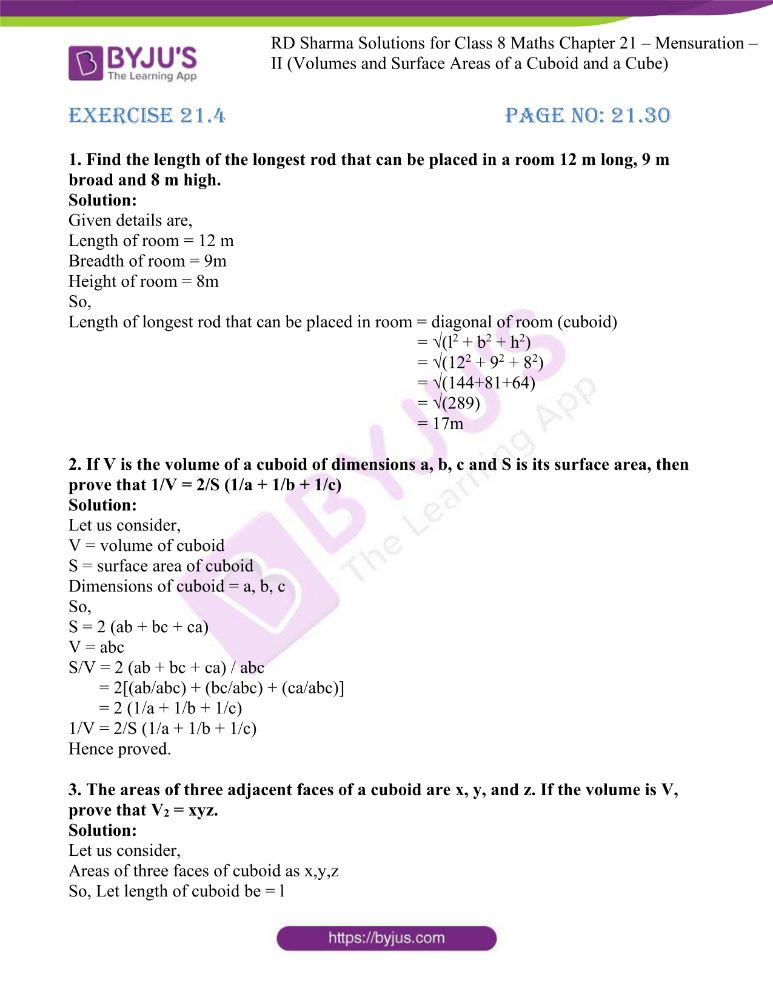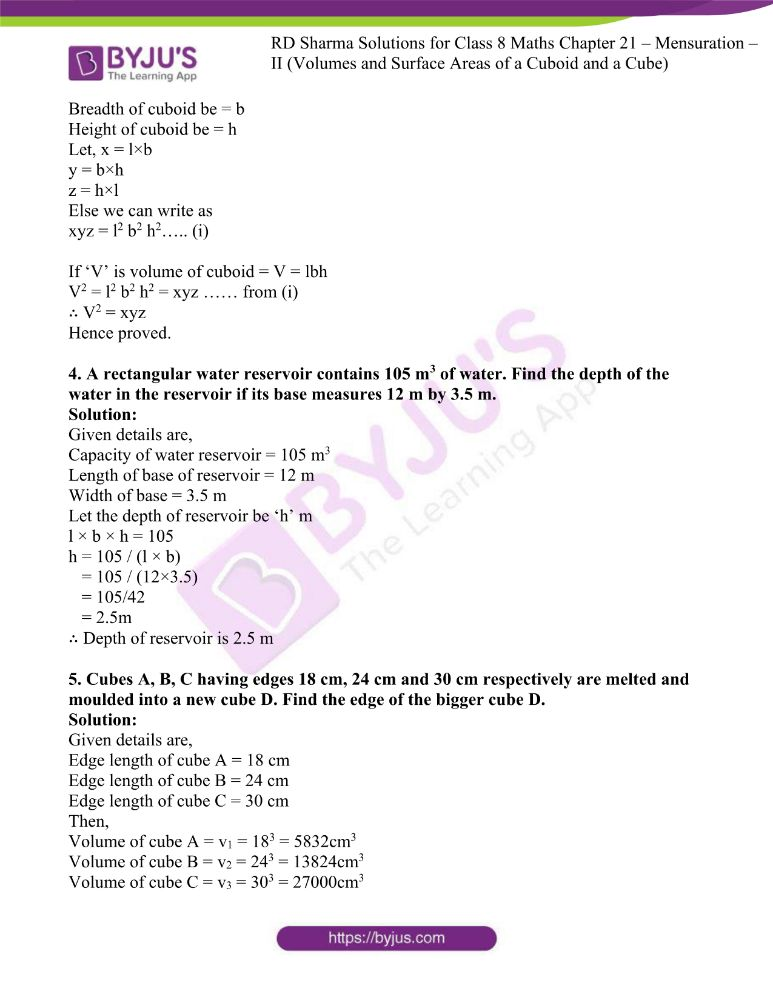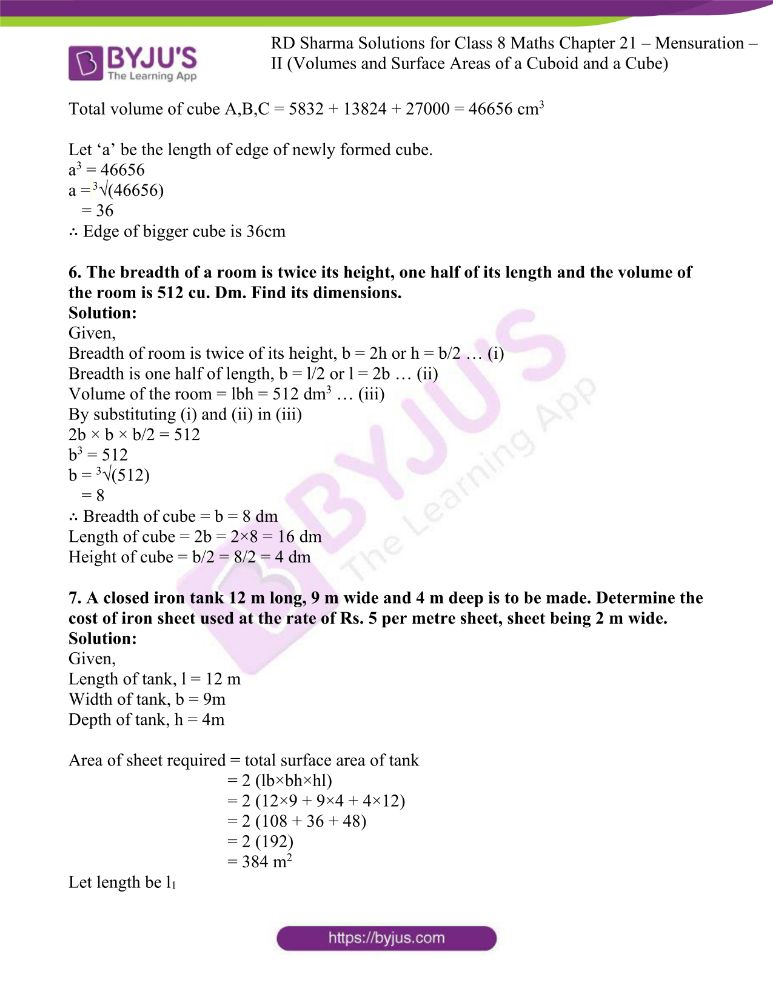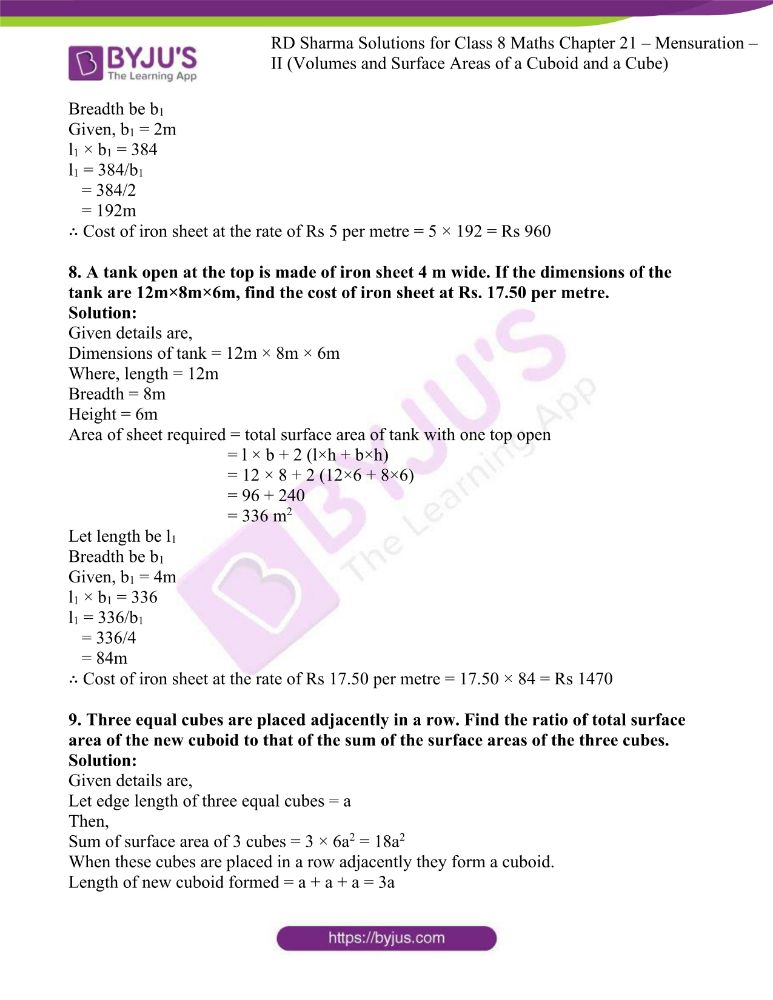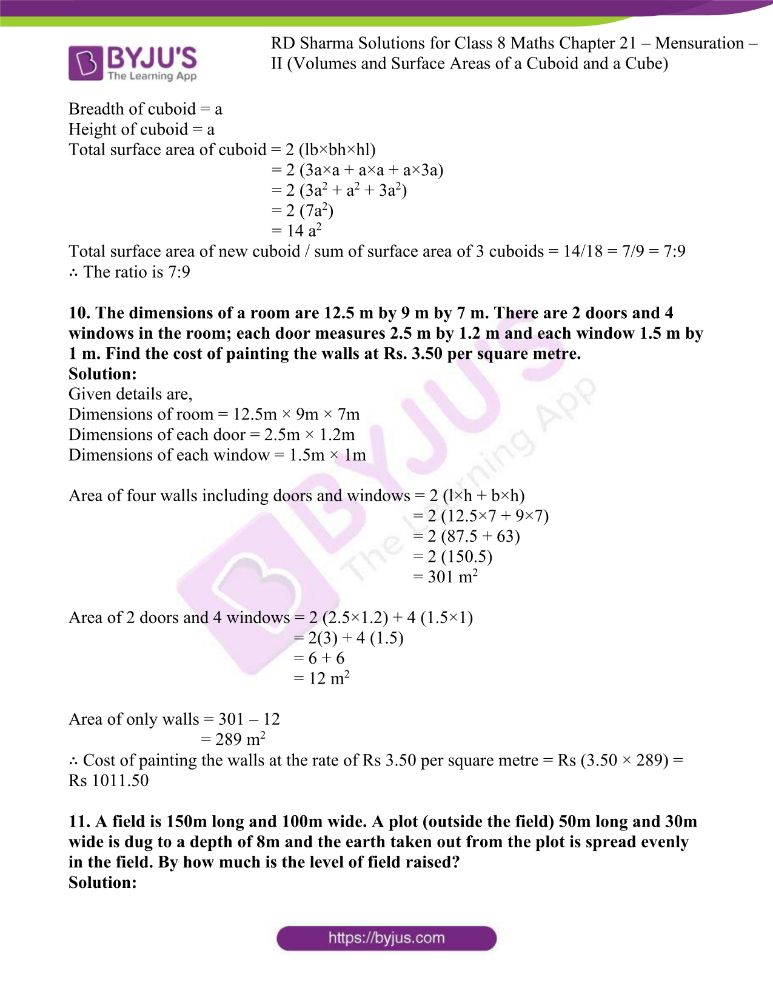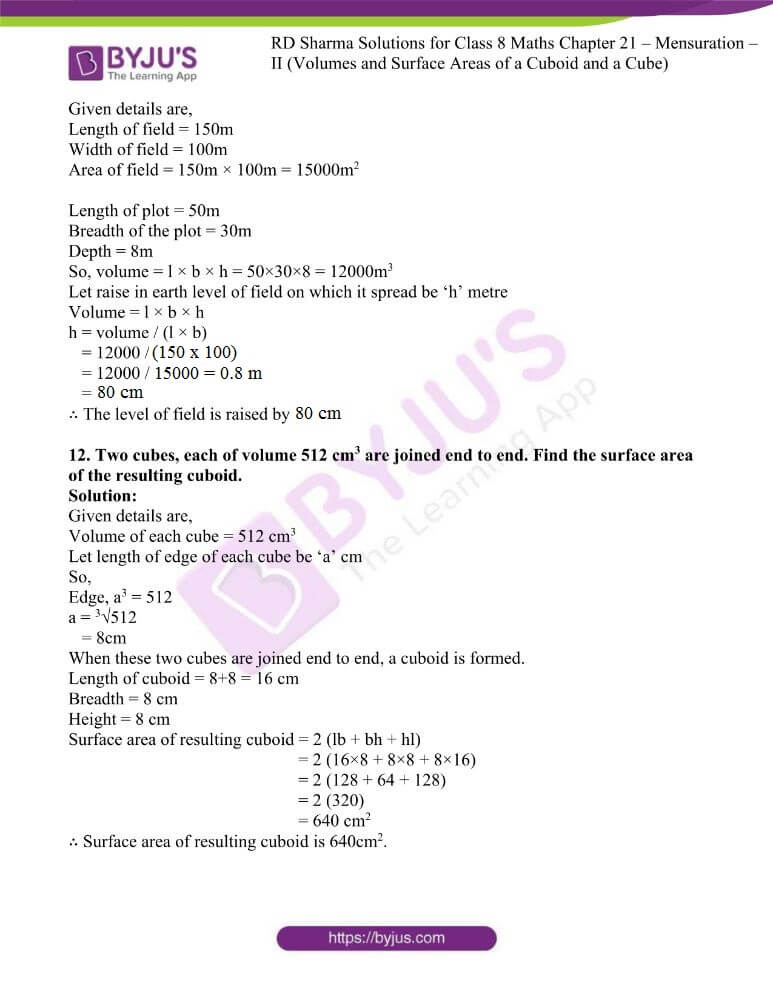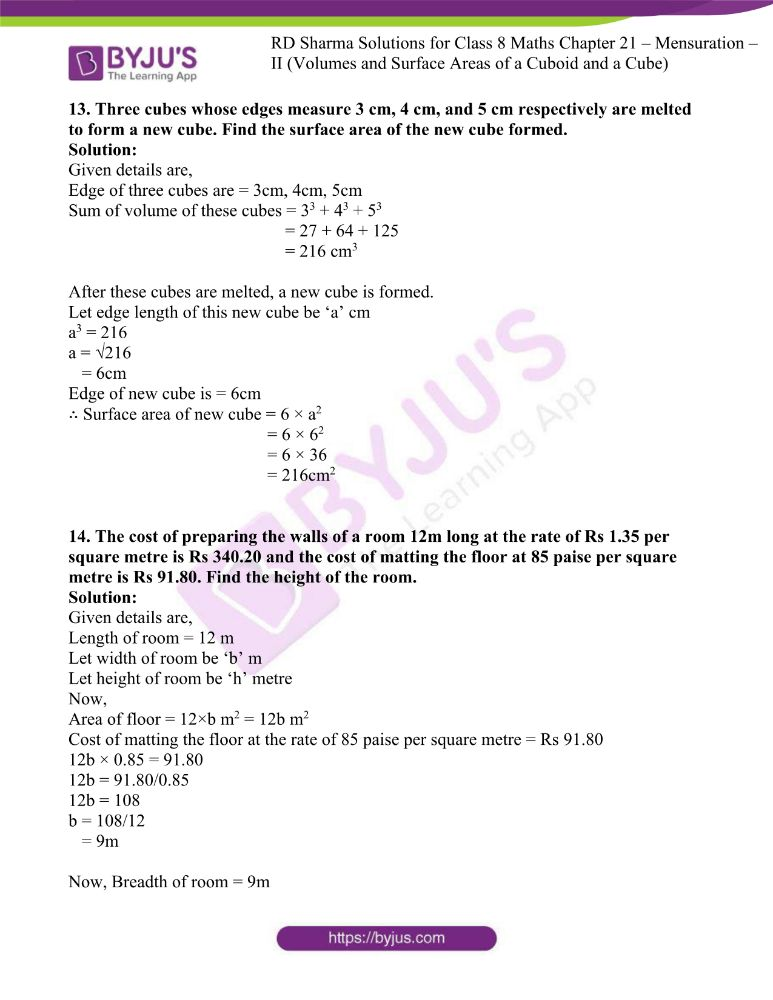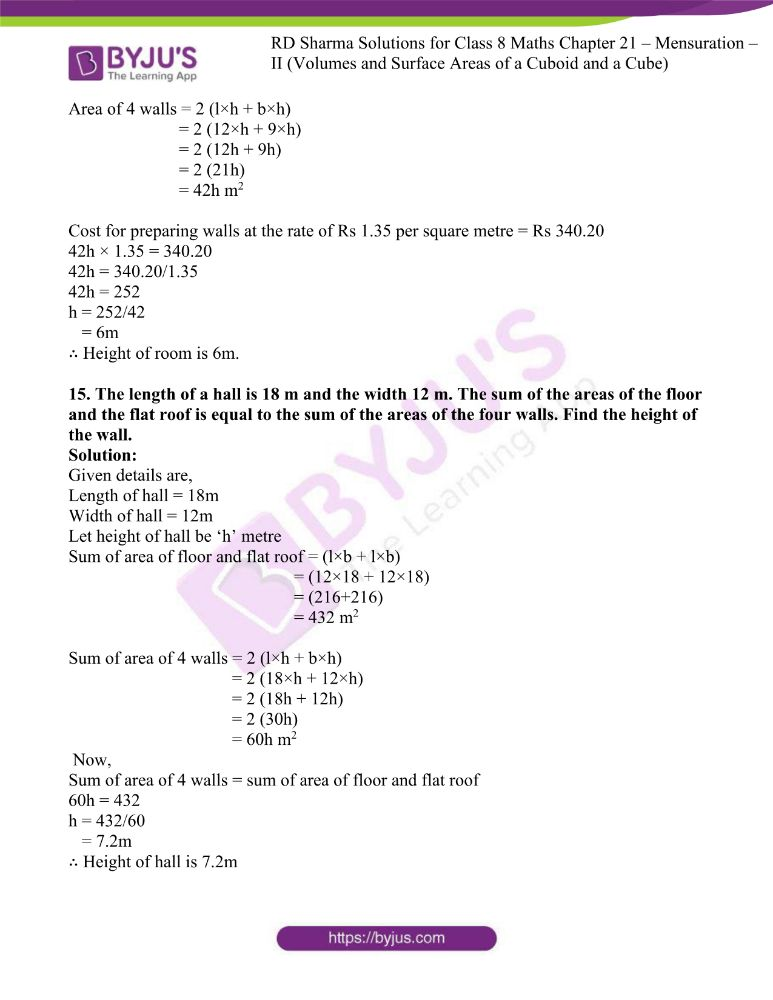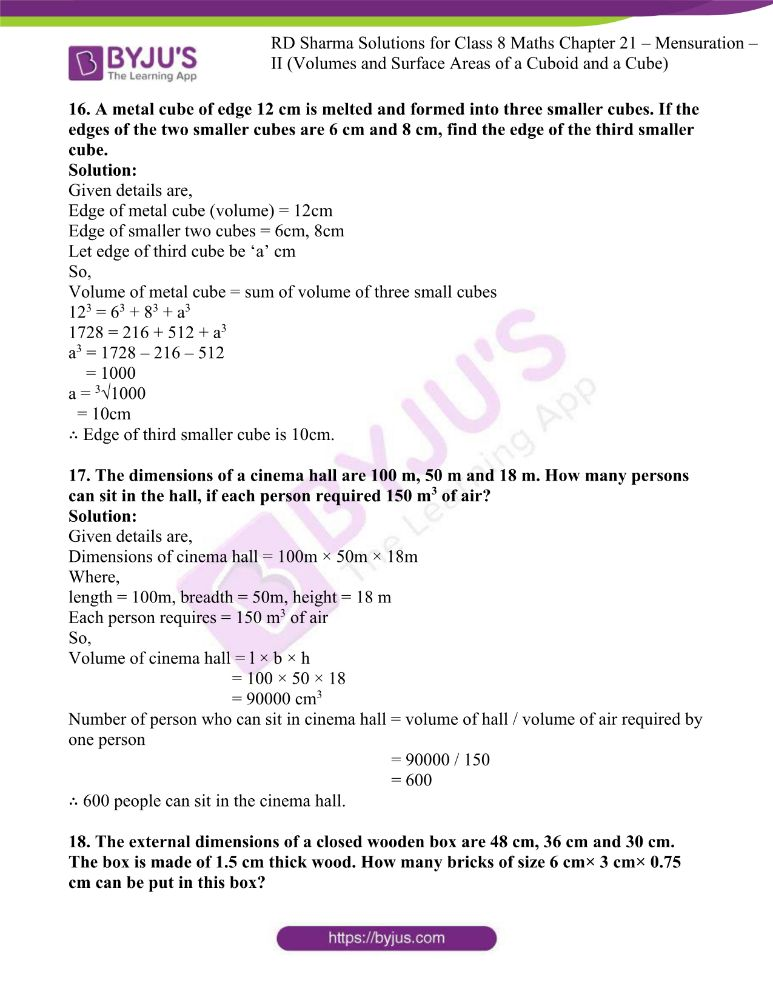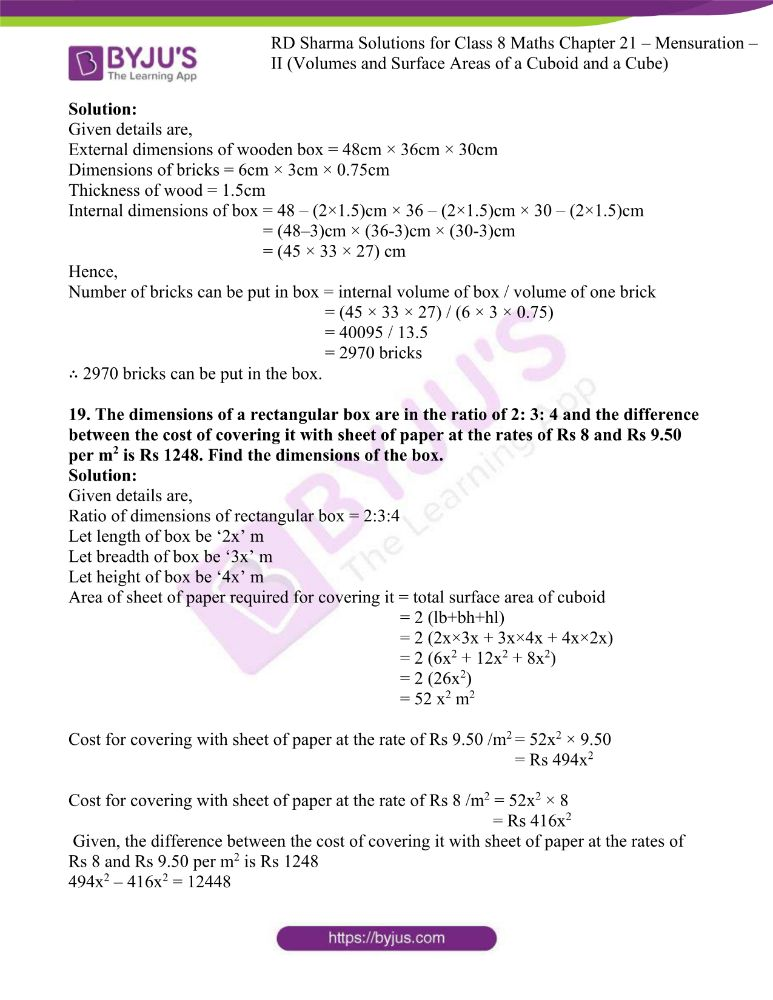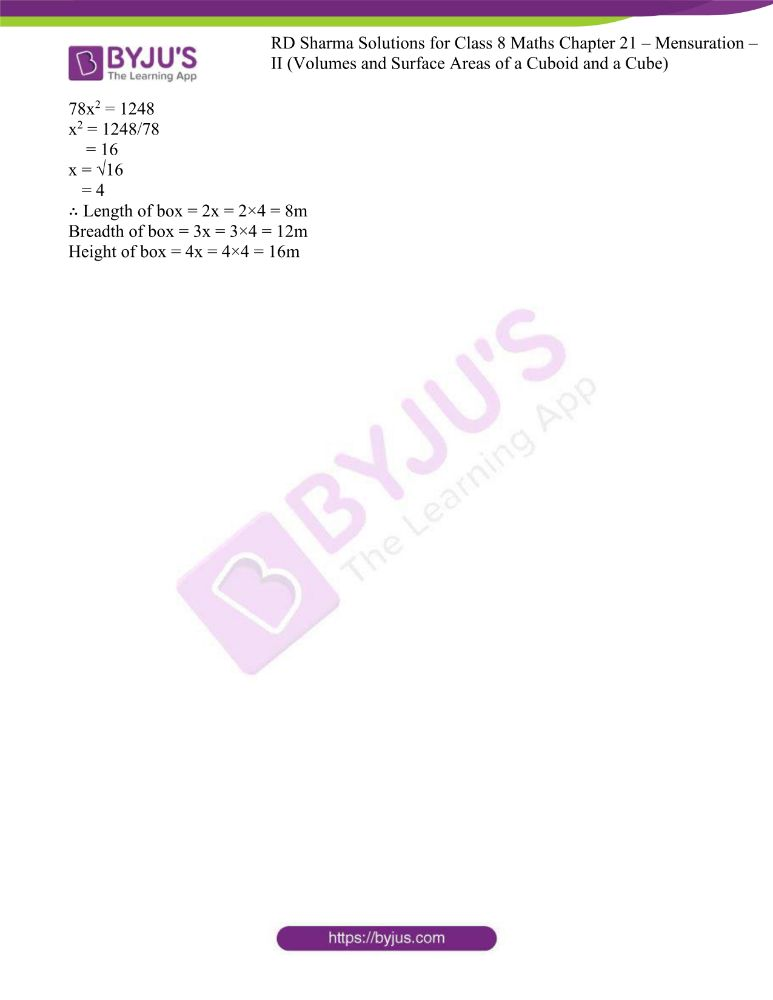### Access answers to RD Sharma Maths Solutions For Class 8 Exercise 21.4 Chapter 21 Mensuration – II (Volumes and Surface Areas of a Cuboid and a Cube)

1. Find the length of the longest rod that can be placed in a room 12 m long, 9 m broad and 8 m high.

Solution:

Given details are,

Length of room = 12 m

Height of room = 8m

So,

Length of longest rod that can be placed in room = diagonal of room (cuboid)

= √(l2 + b2 + h2)

= √(122 + 92 + 82)

= √(144+81+64)

= √(289)

= 17m

2. If V is the volume of a cuboid of dimensions a, b, c and S is its surface area, then prove that 1/V = 2/S (1/a + 1/b + 1/c)

Solution:

Let us consider,

V = volume of cuboid

S = surface area of cuboid

Dimensions of cuboid = a, b, c

So,

S = 2 (ab + bc + ca)

V = abc

S/V = 2 (ab + bc + ca) / abc

= 2[(ab/abc) + (bc/abc) + (ca/abc)]

= 2 (1/a + 1/b + 1/c)

1/V = 2/S (1/a + 1/b + 1/c)

Hence proved.

3. The areas of three adjacent faces of a cuboid are x, y, and z. If the volume is V, prove that V2 = xyz.

Solution:

Let us consider,

Areas of three faces of cuboid as x,y,z

So, Let length of cuboid be = l

Breadth of cuboid be = b

Height of cuboid be = h

Let, x = l×b

y = b×h

z = h×l

Else we can write as

xyz = l2 b2 h2….. (i)

If ‘V’ is volume of cuboid = V = lbh

V2 = l2 b2 h2 = xyz …… from (i)

∴ V2 = xyz

Hence proved.

4. A rectangular water reservoir contains 105 m3 of water. Find the depth of the water in the reservoir if its base measures 12 m by 3.5 m.

Solution:

Given details are,

Capacity of water reservoir = 105 m3

Length of base of reservoir = 12 m

Width of base = 3.5 m

Let the depth of reservoir be ‘h’ m

l × b × h = 105

h = 105 / (l × b)

= 105 / (12×3.5)

= 105/42

= 2.5m

∴ Depth of reservoir is 2.5 m

5. Cubes A, B, C having edges 18 cm, 24 cm and 30 cm respectively are melted and moulded into a new cube D. Find the edge of the bigger cube D.

Solution:

Given details are,

Edge length of cube A = 18 cm

Edge length of cube B = 24 cm

Edge length of cube C = 30 cm

Then,

Volume of cube A = v1 = 183 = 5832cm3

Volume of cube B = v2 = 243 = 13824cm3

Volume of cube C = v3 = 303 = 27000cm3

Total volume of cube A,B,C = 5832 + 13824 + 27000 = 46656 cm3

Let ‘a’ be the length of edge of newly formed cube.

a3 = 46656

a = 3√(46656)

= 36

∴ Edge of bigger cube is 36cm

6. The breadth of a room is twice its height, one half of its length and the volume of the room is 512 cu. Dm. Find its dimensions.

Solution:

Given,

Breadth of room is twice of its height, b = 2h or h = b/2 … (i)

Breadth is one half of length, b = l/2 or l = 2b … (ii)

Volume of the room = lbh = 512 dm3 … (iii)

By substituting (i) and (ii) in (iii)

2b × b × b/2 = 512

b3 = 512

b = 3√(512)

= 8

∴ Breadth of cube = b = 8 dm

Length of cube = 2b = 2×8 = 16 dm

Height of cube = b/2 = 8/2 = 4 dm

7. A closed iron tank 12 m long, 9 m wide and 4 m deep is to be made. Determine the cost of iron sheet used at the rate of Rs. 5 per metre sheet, sheet being 2 m wide.

Solution:

Given,

Length of tank, l = 12 m

Width of tank, b = 9m

Depth of tank, h = 4m

Area of sheet required = total surface area of tank

= 2 (lb×bh×hl)

= 2 (12×9 + 9×4 + 4×12)

= 2 (108 + 36 + 48)

= 2 (192)

= 384 m2

Let length be l1

Given, b1 = 2m

l1 × b1 = 384

l1 = 384/b1

= 384/2

= 192m

∴ Cost of iron sheet at the rate of Rs 5 per metre = 5 × 192 = Rs 960

8. A tank open at the top is made of iron sheet 4 m wide. If the dimensions of the tank are 12m×8m×6m, find the cost of iron sheet at Rs. 17.50 per metre.

Solution:

Given details are,

Dimensions of tank = 12m × 8m × 6m

Where, length = 12m

Height = 6m

Area of sheet required = total surface area of tank with one top open

= l × b + 2 (l×h + b×h)

= 12 × 8 + 2 (12×6 + 8×6)

= 96 + 240

= 336 m2

Let length be l1

Given, b1 = 4m

l1 × b1 = 336

l1 = 336/b1

= 336/4

= 84m

∴ Cost of iron sheet at the rate of Rs 17.50 per metre = 17.50 × 84 = Rs 1470

9. Three equal cubes are placed adjacently in a row. Find the ratio of total surface area of the new cuboid to that of the sum of the surface areas of the three cubes.

Solution:

Given details are,

Let edge length of three equal cubes = a

Then,

Sum of surface area of 3 cubes = 3 × 6a2 = 18a2

When these cubes are placed in a row adjacently they form a cuboid.

Length of new cuboid formed = a + a + a = 3a

Height of cuboid = a

Total surface area of cuboid = 2 (lb×bh×hl)

= 2 (3a×a + a×a + a×3a)

= 2 (3a2 + a2 + 3a2)

= 2 (7a2)

= 14 a2

Total surface area of new cuboid / sum of surface area of 3 cuboids = 14/18 = 7/9 = 7:9

∴ The ratio is 7:9

10. The dimensions of a room are 12.5 m by 9 m by 7 m. There are 2 doors and 4 windows in the room; each door measures 2.5 m by 1.2 m and each window 1.5 m by 1 m. Find the cost of painting the walls at Rs. 3.50 per square metre.

Solution:

Given details are,

Dimensions of room = 12.5m × 9m × 7m

Dimensions of each door = 2.5m × 1.2m

Dimensions of each window = 1.5m × 1m

Area of four walls including doors and windows = 2 (l×h + b×h)

= 2 (12.5×7 + 9×7)

= 2 (87.5 + 63)

= 2 (150.5)

= 301 m2

Area of 2 doors and 4 windows = 2 (2.5×1.2) + 4 (1.5×1)

= 2(3) + 4 (1.5)

= 6 + 6

= 12 m2

Area of only walls = 301 – 12

= 289 m2

∴ Cost of painting the walls at the rate of Rs 3.50 per square metre = Rs (3.50 × 289) = Rs 1011.50

11. A field is 150m long and 100m wide. A plot (outside the field) 50m long and 30m wide is dug to a depth of 8m and the earth taken out from the plot is spread evenly in the field. By how much is the level of field raised?

Solution:

Given details are,

Length of field = 150m

Width of field = 100m

Area of field = 150m × 100m = 15000m2

Length of plot = 50m

Breadth of the plot = 30m

Depth = 8m

So, volume = l × b × h = 50×30×8 = 12000m3

Let raise in earth level of field on which it spread be ‘h’ metre

Volume = l × b × h

h = volume / (l × b)

= 12000 / (150×100)

= 12000 / 15000

= 0.8m

= 80cm

∴ The level of field is raised by 80cm.

12. Two cubes, each of volume 512 cm3 are joined end to end. Find the surface area of the resulting cuboid.

Solution:

Given details are,

Volume of each cube = 512 cm3

Let length of edge of each cube be ‘a’ cm

So,

Edge, a3 = 512

a = 3√512

= 8cm

When these two cubes are joined end to end, a cuboid is formed.

Length of cuboid = 8+8 = 16 cm

Height = 8 cm

Surface area of resulting cuboid = 2 (lb + bh + hl)

= 2 (16×8 + 8×8 + 8×16)

= 2 (128 + 64 + 128)

= 2 (320)

= 640 cm2

∴ Surface area of resulting cuboid is 640cm2.

13. Three cubes whose edges measure 3 cm, 4 cm, and 5 cm respectively are melted to form a new cube. Find the surface area of the new cube formed.

Solution:

Given details are,

Edge of three cubes are = 3cm, 4cm, 5cm

Sum of volume of these cubes = 33 + 43 + 53

= 27 + 64 + 125

= 216 cm3

After these cubes are melted, a new cube is formed.

Let edge length of this new cube be ‘a’ cm

a3 = 216

a = √216

= 6cm

Edge of new cube is = 6cm

∴ Surface area of new cube = 6 × a2

= 6 × 62

= 6 × 36

= 216cm2

14. The cost of preparing the walls of a room 12m long at the rate of Rs 1.35 per square metre is Rs 340.20 and the cost of matting the floor at 85 paise per square metre is Rs 91.80. Find the height of the room.

Solution:

Given details are,

Length of room = 12 m

Let width of room be ‘b’ m

Let height of room be ‘h’ metre

Now,

Area of floor = 12×b m2 = 12b m2

Cost of matting the floor at the rate of 85 paise per square metre = Rs 91.80

12b × 0.85 = 91.80

12b = 91.80/0.85

12b = 108

b = 108/12

= 9m

Now, Breadth of room = 9m

Area of 4 walls = 2 (l×h + b×h)

= 2 (12×h + 9×h)

= 2 (12h + 9h)

= 2 (21h)

= 42h m2

Cost for preparing walls at the rate of Rs 1.35 per square metre = Rs 340.20

42h × 1.35 = 340.20

42h = 340.20/1.35

42h = 252

h = 252/42

= 6m

∴ Height of room is 6m.

15. The length of a hall is 18 m and the width 12 m. The sum of the areas of the floor and the flat roof is equal to the sum of the areas of the four walls. Find the height of the wall.

Solution:

Given details are,

Length of hall = 18m

Width of hall = 12m

Let height of hall be ‘h’ metre

Sum of area of floor and flat roof = (l×b + l×b)

= (12×18 + 12×18)

= (216+216)

= 432 m2

Sum of area of 4 walls = 2 (l×h + b×h)

= 2 (18×h + 12×h)

= 2 (18h + 12h)

= 2 (30h)

= 60h m2

Now,

Sum of area of 4 walls = sum of area of floor and flat roof

60h = 432

h = 432/60

= 7.2m

∴ Height of hall is 7.2m

16. A metal cube of edge 12 cm is melted and formed into three smaller cubes. If the edges of the two smaller cubes are 6 cm and 8 cm, find the edge of the third smaller cube.

Solution:

Given details are,

Edge of metal cube (volume) = 12cm

Edge of smaller two cubes = 6cm, 8cm

Let edge of third cube be ‘a’ cm

So,

Volume of metal cube = sum of volume of three small cubes

123 = 63 + 83 + a3

1728 = 216 + 512 + a3

a3 = 1728 – 216 – 512

= 1000

a = 3√1000

= 10cm

∴ Edge of third smaller cube is 10cm.

17. The dimensions of a cinema hall are 100 m, 50 m and 18 m. How many persons can sit in the hall, if each person required 150 m3 of air?

Solution:

Given details are,

Dimensions of cinema hall = 100m × 50m × 18m

Where,

length = 100m, breadth = 50m, height = 18 m

Each person requires = 150 m3 of air

So,

Volume of cinema hall = l × b × h

= 100 × 50 × 18

= 90000 cm3

Number of person who can sit in cinema hall = volume of hall / volume of air required by one person

= 90000 / 150

= 600

∴ 600 people can sit in the cinema hall.

18. The external dimensions of a closed wooden box are 48 cm, 36 cm and 30 cm. The box is made of 1.5 cm thick wood. How many bricks of size 6 cm× 3 cm× 0.75 cm can be put in this box?

Solution:

Given details are,

External dimensions of wooden box = 48cm × 36cm × 30cm

Dimensions of bricks = 6cm × 3cm × 0.75cm

Thickness of wood = 1.5cm

Internal dimensions of box = 48 – (2×1.5)cm × 36 – (2×1.5)cm × 30 – (2×1.5)cm

= (48–3)cm × (36-3)cm × (30-3)cm

= (45 × 33 × 27) cm

Hence,

Number of bricks can be put in box = internal volume of box / volume of one brick

= (45 × 33 × 27) / (6 × 3 × 0.75)

= 40095 / 13.5

= 2970 bricks

∴ 2970 bricks can be put in the box.

19. The dimensions of a rectangular box are in the ratio of 2: 3: 4 and the difference between the cost of covering it with sheet of paper at the rates of Rs 8 and Rs 9.50 per m2 is Rs 1248. Find the dimensions of the box.

Solution:

Given details are,

Ratio of dimensions of rectangular box = 2:3:4

Let length of box be ‘2x’ m

Let breadth of box be ‘3x’ m

Let height of box be ‘4x’ m

Area of sheet of paper required for covering it = total surface area of cuboid

= 2 (lb+bh+hl)

= 2 (2x×3x + 3x×4x + 4x×2x)

= 2 (6x2 + 12x2 + 8x2)

= 2 (26x2)

= 52 x2 m2

Cost for covering with sheet of paper at the rate of Rs 9.50 /m2 = 52x2 × 9.50

= Rs 494x2

Cost for covering with sheet of paper at the rate of Rs 8 /m2 = 52x2 × 8

= Rs 416x2

Given, the difference between the cost of covering it with sheet of paper at the rates of Rs 8 and Rs 9.50 per m2 is Rs 1248

494x2 – 416x2 = 12448

78x2 = 1248

x2 = 1248/78

= 16

x = √16

= 4

∴ Length of box = 2x = 2×4 = 8m

Breadth of box = 3x = 3×4 = 12m

Height of box = 4x = 4×4 = 16m